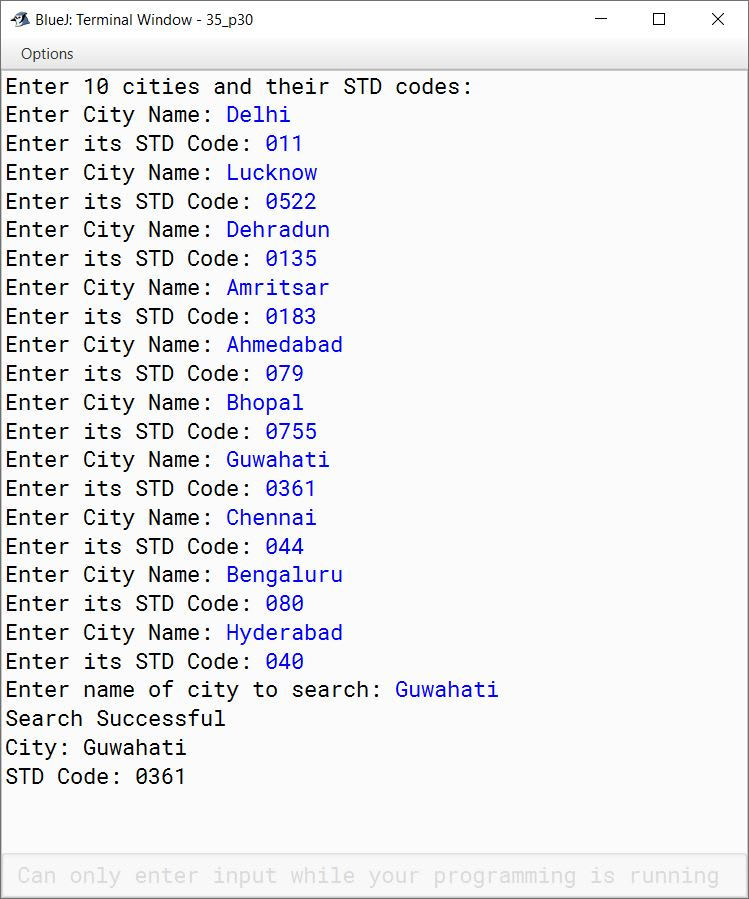# Solved 2012 Question Paper ICSE Class 10 Computer Applications

## Section A

#### Question 1

(a) Give one example each of a primitive data type and a composite data type.

1. int
2. array

(b) Give one point of difference between unary and binary operators.

unary operators operate on a single operand whereas binary operators operate on two operands.

(c) Differentiate between call by value or pass by value and call by reference or pass by reference.

Call by valueCall by reference
Values of actual parameters are copied to formal parameters.Reference of actual parameters is passed to formal parameters.
Changes made to formal parameters are not reflected back to actual parameters.Changes made to formal parameters are reflected back to actual parameters.

(d) Write a Java expression for:

$\sqrt{2as + u^2}$

Math.sqrt(2 * a * s + u * u)

(e) Name the type of error (syntax, runtime or logical error) in each case given below:

1. Division by a variable that contains a value of zero.
2. Multiplication operator used when the operation should be division.
3. Missing semicolon.

1. Runtime error
2. Logical error
3. syntax error

#### Question 2

(a) Create a class with one integer instance variable. Initialize the variable using:

1. default constructor
2. parameterized constructor

class Number {
int a;

public Number() {
a = 0;
}

public Number(int x) {
a = x;
}
}

(b) Complete the code below to create an object of Scanner class:

Scanner sc = ____ Scanner(__________);

Scanner sc = new Scanner(System.in);

(c) What is an array? Write a statement to declare an integer array of 10 elements.

An array is a structure to store a number of values of the same data type in contiguous memory locations. The following statement declares an integer array of 10 elements:

int arr[] = new int;

(d) Name the search or sort algorithm that:

1. Makes several passes through the array, selecting the next smallest item in the array each time and placing it where it belongs in the array.
2. At each stage, compares the sought key value with the key value of the middle element of the array.

1. Selection Sort
2. Binary Search

(e) Differentiate between public and private modifiers for members of a class.

public modifier makes the class members accessible both within and outside their class whereas private modifier makes the class members accessible only within the class in which they are declared.

#### Question 3

(a) What are the values of x and y when the following statements are executed?

int a = 63, b = 36;
boolean x = (a > b)? true : false;
int y = (a < b)? a : b;

##### Output
x = true
y = 36

##### Explanation

The ternary operator (a > b)? true : false returns true as its condition a > b is true so it returns its first expression that is true.

The ternary operator (a < b)? a : b returns b as its condition a < b is false so it returns its second expression that is b. Value of b is 36 so y also becomes 36.

(b) State the values of n and ch.

char c = 'A';
int n = c + 1;
char ch = (char)n;

Value of n is 66 and ch is B.

int n = c + 1, here due to implicit conversion, 'A' will be converted to its ASCII value 65. 1 is added to it making the value of n 66.

char ch = (char)n, here through explicit conversion 66 is converted to its corresponding character that is 'B' so value of ch becomes B.

(c) What will be the result stored in x after evaluating the following expression?

int x = 4;
x += (x++) + (++x) + x;

x += (x++) + (++x) + x
⇒ x = x + ((x++) + (++x) + x)
⇒ x = 4 + (4 + 6 + 6)
⇒ x = 4 + 16
⇒ x = 20

(d) Give output of the following program segment:

double x = 2.9, y = 2.5;
System.out.println(Math.min(Math.floor(x), y));
System.out.println(Math.max(Math.ceil(x), y));

##### Output
2.0
3.0

##### Explanation

Math.floor(2.9) gives 2.0. Math.min(2.0, 2.5) gives 2.0.

Math.ceil(2.9) gives 3.0. Math.max(3.0, 2.5) gives 3.0.

(e) State the output of the following program segment:

String s = "Examination";
int n = s.length();
System.out.println(s.startsWith(s.substring(5, n)));
System.out.println(s.charAt(2) == s.charAt(6));

##### Output
false
true

##### Explanation

Putting the value of n in s.substring(5, n), it becomes s.substring(5, 11). This gives "nation". As s does not start with "nation" so s.startsWith(s.substring(5, n)) returns false.

s.charAt(2) is a and s.charAt(6) is also a so both are equal

(f) State the method that:

1. Converts a string to a primitive float data type.
2. Determines if the specified character is an uppercase character.

1. Float.parseFloat()
2. Character.isUpperCase()

(g) State the data type and values of a and b after the following segment is executed:

String s1 = "Computer", s2 = "Applications"
a = (s1.compareTo(s2));
b = (s1.equals(s2));

Data type of a is int and value is 2. Data type of b is boolean and value is false.

(h) What will the following code output:

String s = "malayalam";
System.out.println(s.indexOf('m'));
System.out.println(s.lastIndexOf('m'));

##### Output
0
8

##### Explanation

The first m appears at index 0 and the last m appears at index 8.

(i) Rewrite the following program segment using while instead of for statement.

int f = 1, i;
for(i = 1; i <= 5; i++){
f *= i;
System.out.println(f);
}

int f = 1, i;
while (i <= 5) {
f *= i;
System.out.println(f);
i++;
}

(j) In the program given below, state the name and the value of the:

1. method argument or argument variable
2. class variable
3. local variable
4. instance variable
class MyClass{
static int x = 7;
int y = 2;
public static void main(String args[]){
MyClass obj = new MyClass();
System.out.println(x);
obj.sampleMethod(5);
int a = 6;
System.out.println(a);
}
void sampleMethod(int n){
System.out.println(n);
System.out.println(y);
}
}

1. Method argument/Argument variable is n
2. Class variable is x
3. Local variable is a
4. Instance variable is y

## Section B

#### Question 4

Define a class called Library with the following description:

Instance Variables/Data Members:
int accNum — stores the accession number of the book.
String title — stores the title of the book.
String author — stores the name of the author.

Member methods:

1. void input() — To input and store the accession number, title and author.
2. void compute() — To accept the number of days late, calculate and display the fine charged at the rate of Rs. 2 per day.
3. void display() — To display the details in the following format:
Accession Number    Title   Author


Write a main method to create an object of the class and call the above member methods.

import java.util.Scanner;

public class Library
{
private int accNum;
private String title;
private String author;

void input() {
Scanner in = new Scanner(System.in);
System.out.print("Enter book title: ");
title = in.nextLine();
System.out.print("Enter author: ");
author = in.nextLine();
System.out.print("Enter accession number: ");
accNum = in.nextInt();
}

void compute() {
Scanner in = new Scanner(System.in);
System.out.print("Enter number of days late: ");
int days = in.nextInt();
int fine = days * 2;
System.out.println("Fine = Rs." + fine);
}

void display() {
System.out.println("Accession Number\tTitle\tAuthor");
System.out.println(accNum + "\t\t" + title + "\t" + author);
}

public static void main(String args[]) {
Library obj = new Library();
obj.input();
obj.display();
obj.compute();
}
}
##### Output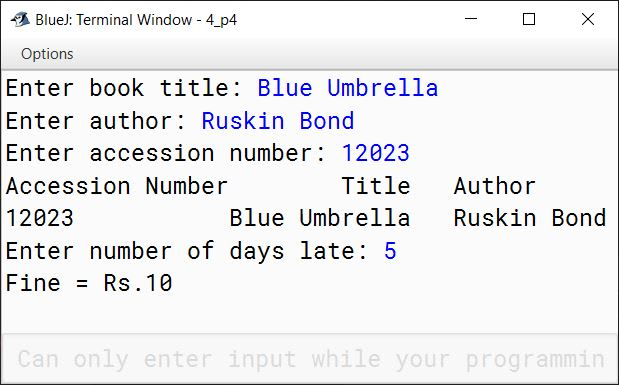#### Question 5

Given below is a hypothetical table showing rates of income tax for male citizens below the age of 65 years:

Taxable income (TI) in ₹Income Tax in ₹
Does not exceed Rs. 1,60,000Nil
Is greater than Rs. 1,60,000 and less than or equal to Rs. 5,00,000.(TI - 1,60,000) x 10%
Is greater than Rs. 5,00,000 and less than or equal to Rs. 8,00,000[(TI - 5,00,000) x 20%] + 34,000
Is greater than Rs. 8,00,000[(TI - 8,00,000) x 30%] + 94,000

Write a program to input the age, gender (male or female) and Taxable Income of a person.

If the age is more than 65 years or the gender is female, display “wrong category”. If the age is less than or equal to 65 years and the gender is male, compute and display the income tax payable as per the table given above.

import java.util.Scanner;

public class KboatIncomeTax
{
public static void main(String args[]) {
Scanner in = new Scanner(System.in);
System.out.print("Enter Gender(male/female): ");
String gender = in.nextLine();
System.out.print("Enter Age: ");
int age = in.nextInt();
System.out.print("Enter Taxable Income: ");
double ti = in.nextDouble();
double tax = 0.0;

if (age > 65 || gender.equalsIgnoreCase("female")) {
System.out.print("Wrong Category");
}
else {
if (ti <= 160000)
tax = 0;
else if (ti <= 500000)
tax = (ti - 160000) * 10 / 100;
else if (ti <= 800000)
tax = 34000 + ((ti - 500000) * 20 / 100);
else
tax = 94000 + ((ti - 800000) * 30 / 100);

System.out.println("Tax Payable: " + tax);
}
}
}
##### Output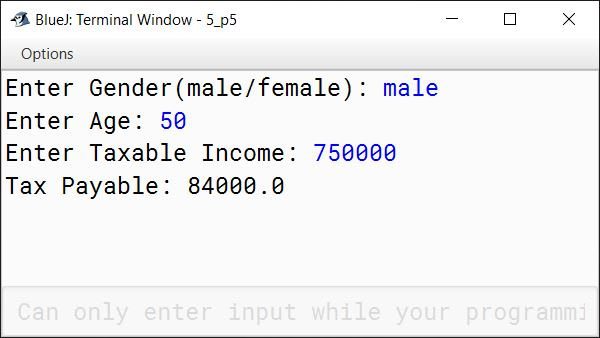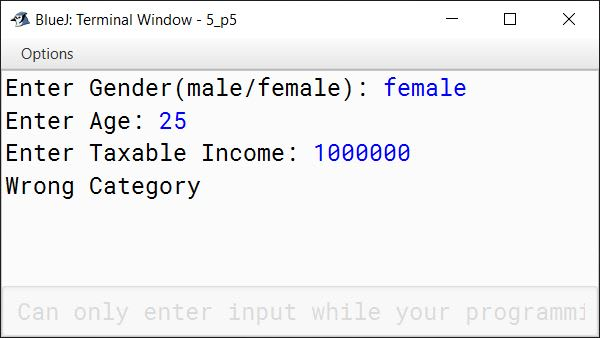#### Question 6

Write a program to accept a string. Convert the string into upper case letters. Count and output the number of double letter sequences that exist in the string.
Sample Input: "SHE WAS FEEDING THE LITTLE RABBIT WITH AN APPLE"
Sample Output: 4

import java.util.Scanner;

public class KboatLetterSeq
{
public static void main(String args[]) {
Scanner in = new Scanner(System.in);
System.out.print("Enter string: ");
String s = in.nextLine();
String str = s.toUpperCase();
int count = 0;
int len = str.length();

for (int i = 0; i < len - 1; i++) {
if (str.charAt(i) == str.charAt(i + 1))
count++;
}

System.out.println("Double Letter Sequence Count = " + count);

}
}
##### Output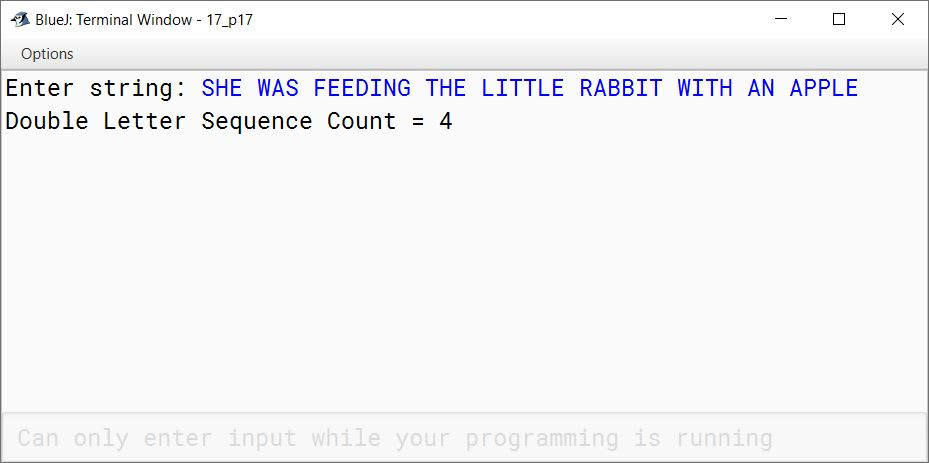#### Question 7

Design a class to overload a function polygon() as follows:

1. void polygon(int n, char ch) — with one integer and one character type argument to draw a filled square of side n using the character stored in ch.
2. void polygon(int x, int y) — with two integer arguments that draws a filled rectangle of length x and breadth y, using the symbol '@'.
3. void polygon() — with no argument that draws a filled triangle shown below:

Example:

1. Input value of n=2, ch = 'O'
Output:
OO
OO
2. Input value of x = 2, y = 5
Output:
@@@@@
@@@@@
3. Output:
*
**
***

public class KboatPolygon
{
public void polygon(int n, char ch) {
for (int i = 1; i <= n; i++) {
for (int j = 1; j <= n; j++) {
System.out.print(ch);
}
System.out.println();
}
}

public void polygon(int x, int y) {
for (int i = 1; i <= x; i++) {
for (int j = 1; j <= y; j++) {
System.out.print('@');
}
System.out.println();
}
}

public void polygon() {
for (int i = 1; i <= 3; i++) {
for (int j = 1; j <= i; j++) {
System.out.print('*');
}
System.out.println();
}
}

public static void main(String args[]) {
KboatPolygon obj = new KboatPolygon();
obj.polygon(2, 'o');
System.out.println();
obj.polygon(2, 5);
System.out.println();
obj.polygon();
}
}
##### Output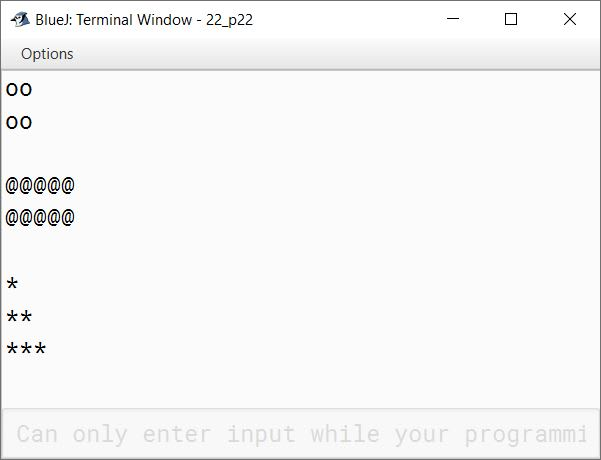#### Question 8

Using a switch statement, write a menu driven program to:
(a) Generate and display the first 10 terms of the Fibonacci series
0, 1, 1, 2, 3, 5
The first two Fibonacci numbers are 0 and 1, and each subsequent number is the sum of the previous two.
(b) Find the sum of the digits of an integer that is input.
Sample Input: 15390
Sample Output: Sum of the digits = 18
For an incorrect choice, an appropriate error message should be displayed.

import java.util.Scanner;

public class KboatFibonacciNDigitSum
{
public static void main(String args[]) {
Scanner in = new Scanner(System.in);
System.out.println("1. Fibonacci Series");
System.out.println("2. Sum of digits");
int ch = in.nextInt();

switch (ch) {
case 1:
int a = 0, b = 1;
System.out.print(a + " " + b);
for (int i = 3; i <= 10; i++) {
int term = a + b;
System.out.print(" " + term);
a = b;
b = term;
}
break;

case 2:
System.out.print("Enter number: ");
int num = in.nextInt();
int sum = 0;
while (num != 0) {
sum += num % 10;
num /= 10;
}
System.out.println("Sum of Digits " + " = " + sum);
break;

default:
System.out.println("Incorrect choice");
break;
}
}
}
##### Output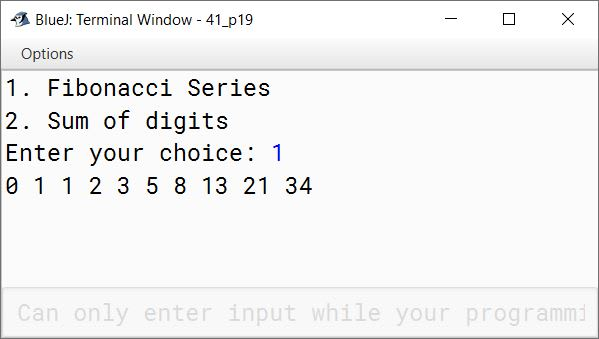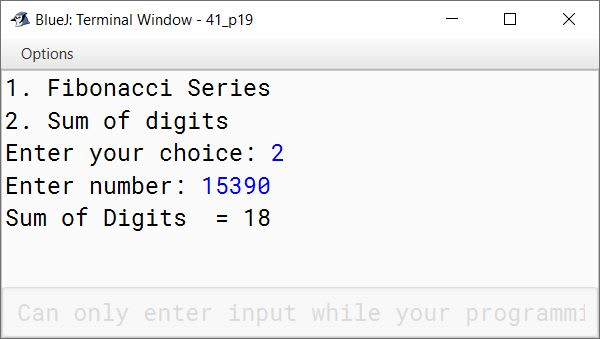#### Question 9

Write a program to accept the names of 10 cities in a single dimensional string array and their STD (Subscribers Trunk Dialling) codes in another single dimension integer array. Search for the name of a city input by the user in the list. If found, display "Search Successful" and print the name of the city along with its STD code, or else display the message "Search unsuccessful, no such city in the list".

import java.util.Scanner;

public class KboatStdCodes
{
public static void main(String args[]) {
final int SIZE = 10;
Scanner in = new Scanner(System.in);
String cities[] = new String[SIZE];
String stdCodes[] = new String[SIZE];
System.out.println("Enter " + SIZE +
" cities and their STD codes:");

for (int i = 0;  i < SIZE; i++) {
System.out.print("Enter City Name: ");
cities[i] = in.nextLine();
System.out.print("Enter its STD Code: ");
stdCodes[i] = in.nextLine();
}

System.out.print("Enter name of city to search: ");
String city = in.nextLine();

int idx;
for (idx = 0;  idx < SIZE; idx++) {
if (city.compareToIgnoreCase(cities[idx]) == 0) {
break;
}
}

if (idx < SIZE) {
System.out.println("Search Successful");
System.out.println("City: " + cities[idx]);
System.out.println("STD Code: " + stdCodes[idx]);
}
else {
System.out.println("Search Unsuccessful");
}
}
}
##### Output